﻿ Introduction to Neural Networks Part II – BMC Blogs
Machine Learning & Big Data Blog

# Introduction to Neural Networks Part II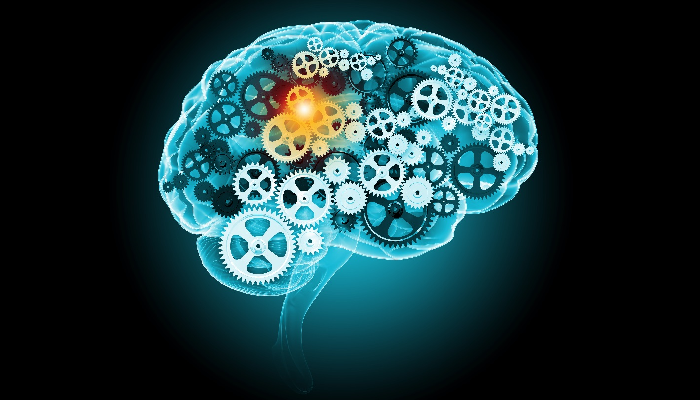In the previous post we introduced the concept of perceptrons, which take inputs from simple linear equations and output 1 (true) or 0 (false). They are the left-hand side of the neural network.But as Michael Nielsen explains, in his book, perceptrons are not suitable for tasks like image recognition because small changes to the weights and biases product large changes to the output. After all, going to 0 to 1 is a large change. It would be better to go from, say, 0.6 to 0.65.

Suppose have a simple neural network with two input variables x1 and x2 and a bias of 3 with weights of -2 and -3. The equation for that is:

If -2×1 + -3×2 + 3 < 0 then 1 (true) otherwise 0 (false).

(That’s not exactly the correct way to express that in algebra, but it is close enough. The goal here is to keep the math to a minimum to make it easier to understand. Michael’s paper is difficult to understand for those without a math background.)

Machine learning adjusts the weights and the biases until the resulting formula most accurately calculates the correct value. Remember from the last post, that this is the same as saying that adjusting the weights and biases reduces the loss function to its minimum. Most ML problems work that way. For example, linear regression.

So how do we avoid the large change of going from 0 to 1, which would mess up our model? We allow inputs and output numbers between 0 and 1 instead of just 0 or 1.

The simplest way to do that is to divide the equation into the number 1, by using a similar formula, as that used by logistic regression. And then we adopt the convention that if the final output value of the neural network has a threshold, say 0.5, then we can conclude that the outcome is 1.

But isn’t that just a roundabout way of calculating something that results in either 0 or 1? No. Because in a neural network there is not just the input initial values and the resulting output. In the middle, there are intermediate steps called hidden layers. Those need not evaluate to 0 or 1.

(You can play around with a neural network to add or remove hidden layers using this online tool.)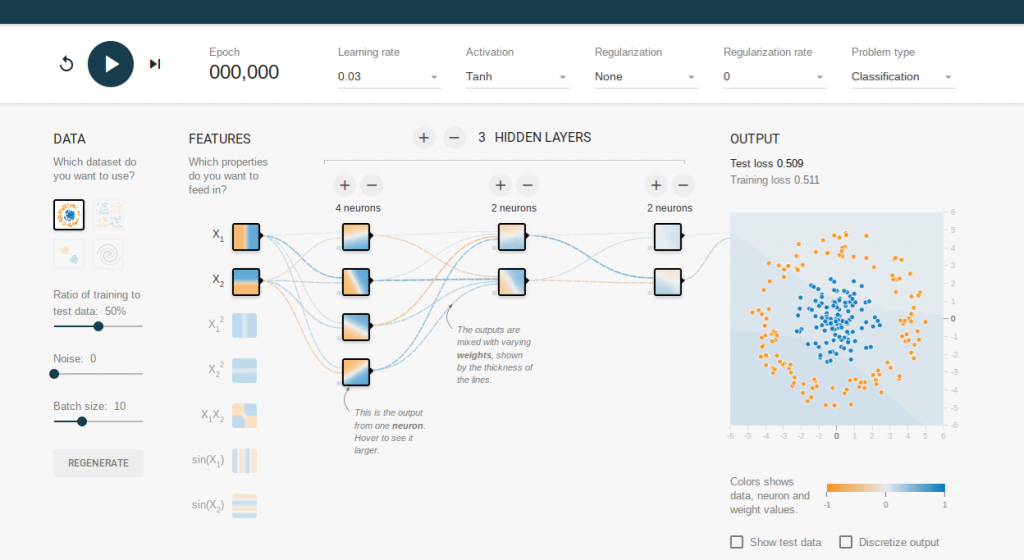To illustrate, let z=x1w1 + x2w2 + b be the function above. Then we create a modified perception called a sigmoid neuron function (δ) like this.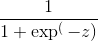Now we state that the values of x1 and x2 in function z do not have to be integers. They can be any value between 0 and 1, as a result of which the sigmoid neuron function δ will vary between 0 and 1.

Remember that exp,the constant e = 2.714. Raising it to a negative power is the same as dividing it into 1, i.e. exp(-z) = 1 / exp(z).

When the value of z is large then exp(-z) is small (close to zero). Because 1 divided by something large is small. In that case, the sigmoid neuron function is close to 1. Conversely, when z is small then 1/(1 + exp(-z) is close to 0. But for values that are neither large nor small, δ does not vary much.

With artificial intelligence, we train the neural network by varying the weights x1, x2, x3, … , xn and the bias b. That is to say, we vary the inputs to minimize the loss function. That is no different than simple linear regression.

Remember that the loss function is just the difference between the predicted value and the observed value. When there is just 1 or 2 inputs that is easy. But with handwriting recognition there are hundreds or thousands of inputs.

(For an image of 256 pixels there are 256 * 256 inputs in our neural network, it looks something like this, except that this has been made smaller so that you can visualize it. And this network only looks at digits and not the whole alphabet.)With simple linear regression, the loss function is the distance between the observed value z and the predicted value p, or z – p. With neural networks we use something more complicated called the stochastic gradient descent, which is not necessary to be understood.It will suffice to say that it is basically the same thing. But finding the minimum value in some function with thousands of input variables is hard to achieve, so the stochastic gradient descent first takes a guess and then works from there.

Michael Nielsen gives this analogy. Below is a graph of a loss function f(x,y), i.e. a function with two inputs. If you drop a marble into that bowl then it will roll to the lowest point. The stochastic gradient descent is an algorithm to find that point for a loss function with many input variables. (For those who know calculus, you might say why not just take the derivative of that function and find its minimum? The answer is that you cannot easily find the derivative for a function with thousands of variables.)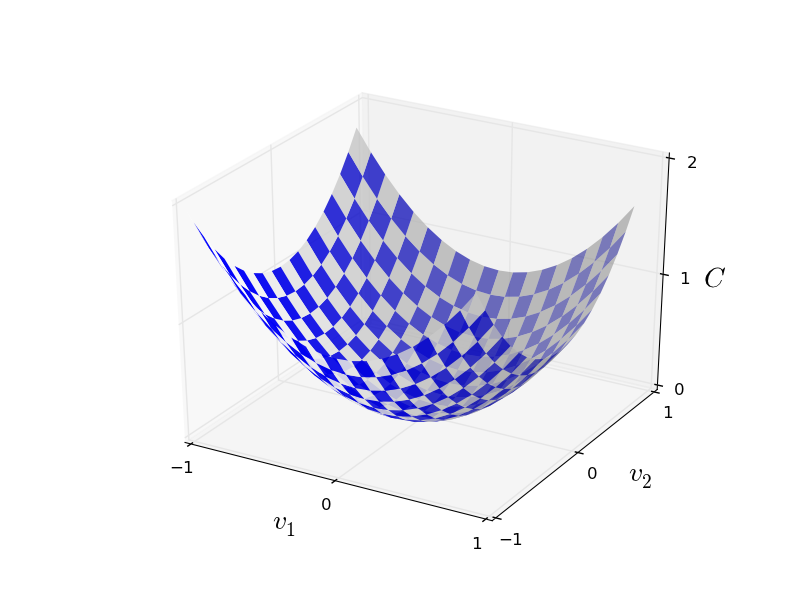Anyway, let’s now see how this works with handwriting recognition. Here is an image of the number “0”. The neural network looks at each pixel, and how dark the pixel is, to figure out which pixels are filled in. Then it matches that with handwriting samples known to represent the number 0.

The MNIST training set takes handwriting samples from 250 people. This data takes the combination of pixels of each drawing and indicates whether it is a 0, 1, 2, …, or 9.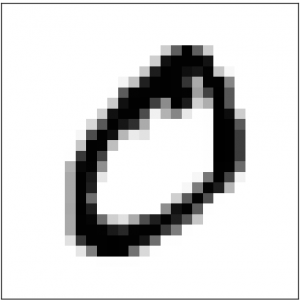The neural network is then trained, based on this data, i.e., it adjusts the coefficients and bias until it most accurately determines what digit it is.

Then you plug in handwriting samples from people who are not present in the training set. This new set of data is called the testing set, which makes it possible to read what these people have written.

### Automate workflows to simplify your big data lifecycle

In this e-book, you’ll learn how you can automate your entire big data lifecycle from end to end—and cloud to cloud—to deliver insights more quickly, easily, and reliably.

These postings are my own and do not necessarily represent BMC's position, strategies, or opinion.

See an error or have a suggestion? Please let us know by emailing blogs@bmc.com.